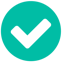## Property Calculation based on other properties.

SOLVEParticipant

Hi everyone,

I would like to create a new property in the Sales Hub which is a calculation based on other properties that prospects can fill in on a landingpage.

Basically, it is a score (in percentage) to see how much efficiency the prospects are losing with their current workflow. Right now, I am doing it manually in Excel but would like to automate it in Hubspot.

The formula that I'm using in Excel is as follows (of course it is adapted for clarity):
=MAX(10%; MIN(80%; 100%-((1-(Input 1/100)) * (1-(Input 2/100)) * (1-(Input 3/1000)))))

I know I cannot have the score in percentage but that I can create properties for 'Input' and can make calculations based on that. However, I am struggling with the rest of the calculation.

Does someone have some good advice?

2 Accepted solutions

Accepted SolutionsSolution
Hall of Famer

Hi @RLV2525,

Does your MIN and MAX formula apply to multiple values submitted by one contact or one value submitted by multiple contacts? HubSpot does have MIN and MAX functions but not for multiple values in one record, see for example the definition of MIN: "displays the minimum value for the selected number property on all associated records within the type selected" (see here)

If you're looking for a function like this, that calculation would have to happen outside of HubSpot, e.g. pushing the data into a spreadsheet (Microsoft Excel, Google Sheets) via Zapier and then transferring the calculated values back into HubSpot.

If I'm misunderstanding your request, generally, the solution would be in nesting multiple calculated properties if it can't be done within one.

Hope this helps!Karsten KöhlerDigital Marketer | HubSpot Freelancer | CRM ConsultantBeratungstermin mit Karsten vereinbarenDid my post help answer your query? Help the community by marking it as a solution.Solution
Participant

Hi @karstenkoehler ,

The problem is solved. It was quite a bypass within Hubspot, but I have done the following:

Property First Calculation Input one:
Calculation: Input 1 / 100
Property Second Calculation Input two:
Calculation: 1 - Calculation input 1

Done that for all the 3 inputs and then did the following:

Property First Calculation All Inputs:
Calculation: Input 1 * Input 2 * Input 3
Property Second Calculation All Inputs:
Calculation: 1 - Calculation All Inputs.

Then I used the MAX & MIN function within Hubspot, and with those results, I made a calculation with that value * 100 to get a "percentage".

Quite some work but it got the result done. Hope it might be of use for you as well in future cases.

Thanks and kind regards,

2 Replies 2Solution
Hall of Famer

Hi @RLV2525,

Does your MIN and MAX formula apply to multiple values submitted by one contact or one value submitted by multiple contacts? HubSpot does have MIN and MAX functions but not for multiple values in one record, see for example the definition of MIN: "displays the minimum value for the selected number property on all associated records within the type selected" (see here)

If you're looking for a function like this, that calculation would have to happen outside of HubSpot, e.g. pushing the data into a spreadsheet (Microsoft Excel, Google Sheets) via Zapier and then transferring the calculated values back into HubSpot.

If I'm misunderstanding your request, generally, the solution would be in nesting multiple calculated properties if it can't be done within one.

Hope this helps!Karsten KöhlerDigital Marketer | HubSpot Freelancer | CRM ConsultantBeratungstermin mit Karsten vereinbarenDid my post help answer your query? Help the community by marking it as a solution.Solution
Participant

Hi @karstenkoehler ,

The problem is solved. It was quite a bypass within Hubspot, but I have done the following:

Property First Calculation Input one:
Calculation: Input 1 / 100
Property Second Calculation Input two:
Calculation: 1 - Calculation input 1

Done that for all the 3 inputs and then did the following:

Property First Calculation All Inputs:
Calculation: Input 1 * Input 2 * Input 3
Property Second Calculation All Inputs:
Calculation: 1 - Calculation All Inputs.

Then I used the MAX & MIN function within Hubspot, and with those results, I made a calculation with that value * 100 to get a "percentage".

Quite some work but it got the result done. Hope it might be of use for you as well in future cases.

Thanks and kind regards,